# Residual Plots

We still use the savings dataset as an example again:

> g <- lm(sav ~ p15 + p75 + inc + gro, data=savings) # fit the model with sav as the response and the rest variables as predictors

Let's plot

• raw residuals,

• studentized residuals, and

• jacknife residuals,

and check if their relative magnitudes are different or similar.

> rstud <- rstandard(g) # studentized residuals
> rjack <- rstudent(g) # jacknife residuals
> par(mfrow=c(2,2)) # split the graphic window into 2x2 subwindows

> plot(g\$res,ylab="raw residuals"); plot(rstud,ylab="studentized residuals"); plot(rjack,ylab="jacknife residuals") # draw raw, studentized, jacknife residuals against index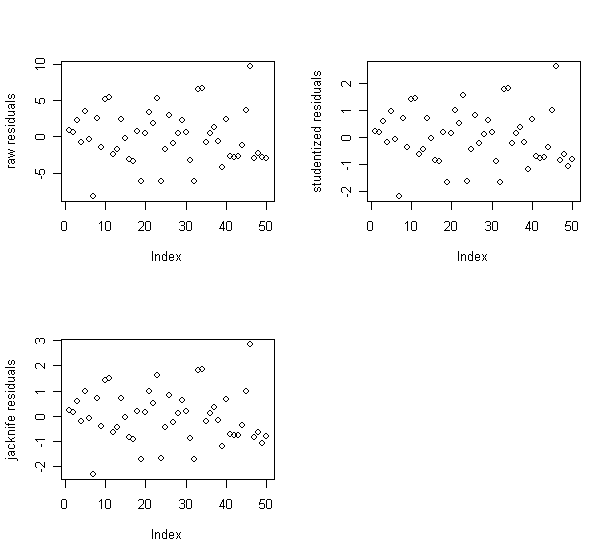• The patterns of the three residual plots look similar.

• Therefore, I decide to use the raw residuals in the following analysis.

First, the residuals vs. fitted plot and the abs(residuals) vs. fitted plot.

> par(mfrow=c(1,2))  # split the graphic window into 1x2 subwindows

> plot(g\$fit, g\$res, xlab="Fitted", ylab="Residuals"); abline(h=0) # draw the plot of residuals against y-hat
> plot(g\$fit,abs(g\$res),xlab="Fitted",ylab="|Residuals|") # draw the plot of absolute residual againt y-hat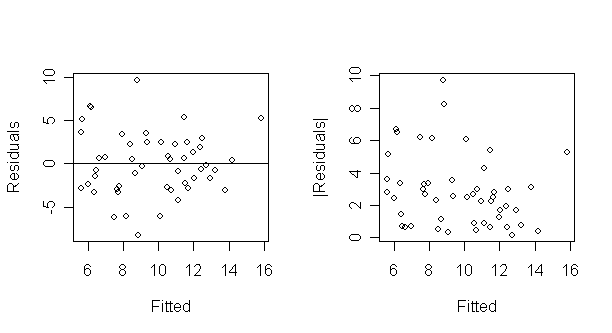• What do you see?

• The latter plot is designed to check for non-constant variance only.

• It folds over the bottom half of the first plot to increase the resolution for detecting non-constant variance.

• A quick way to check non-constant variance is this regression:

> summary(lm(abs(g\$res) ~ g\$fit)) # fit a model with absolute residual as response and y-hat as predictor

Coefficients:

Estimate Std. Error t value Pr(>|t|)

(Intercept)   4.8397     1.1865   4.079 0.000170 ***

g\$fit        -0.2035     0.1185  -1.717 0.092506 .

---

Signif. codes:  0 `***' 0.001 `**' 0.01 `*' 0.05 `.' 0.1 ` ' 1

Residual standard error: 2.163 on 48 degrees of freedom

Multiple R-Squared: 0.05784,    Adjusted R-squared: 0.03821

F-statistic: 2.947 on 1 and 48 DF,  p-value: 0.0925

> abline(summary(lm(abs(g\$res) ~ g\$fit))) # add the fitted line to the absolute residual plot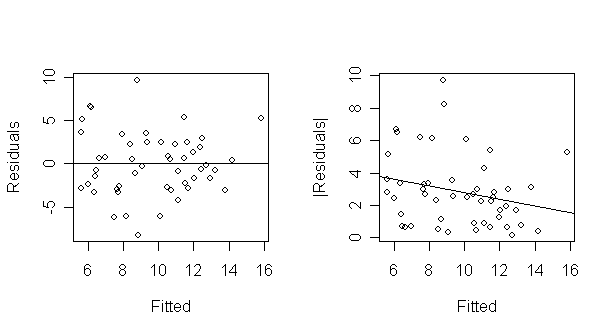• This test is not quite right as some weighting should be used and the degrees of freedom should be adjusted but there doesn't seem to be a clear problem with non-constant variance.

• The former plot is still needed because non-linearity must be checked.

It's often had to judge residual plots without prior experience so let's generate some of the artificial variety. The following four panels show

1. Constant Variance

2. Strong non-constant variance

3. Mild non-constant variance

4. Non-linearity

> par(mfrow=c(3,3)) # split the graphic window into 2x2 subwindows
> for(i in 1:9) {plot(1:50,rnorm(50)); abline(h=0)} # Constant Variance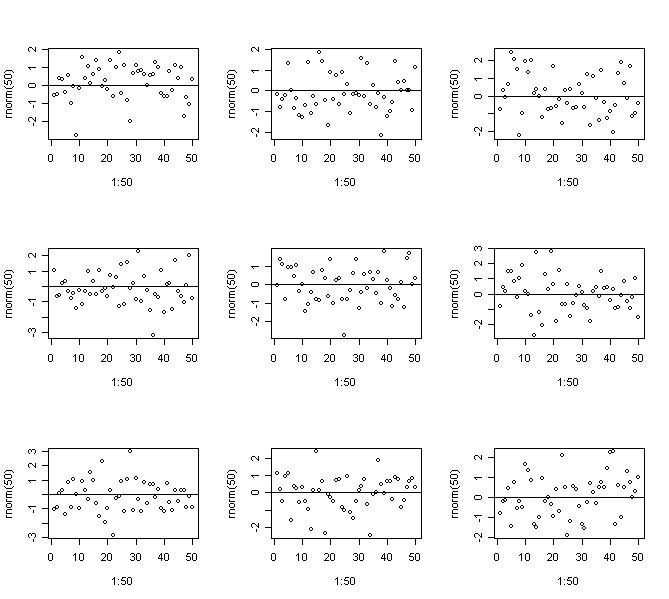> for(i in 1:9) {plot(1:50,(1:50)*rnorm(50)); abline(h=0)}  # Strong non-constant variance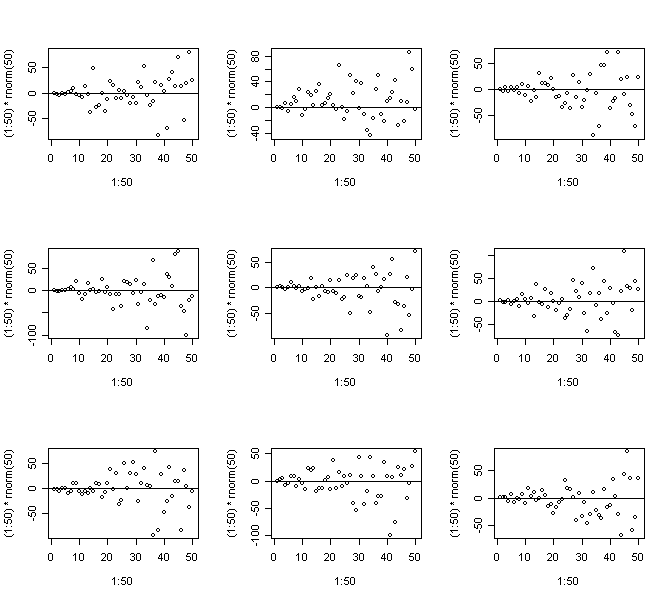> for(i in 1:9) {plot(1:50,sqrt((1:50))*rnorm(50)); abline(h=0)}  # Mild non-constant variance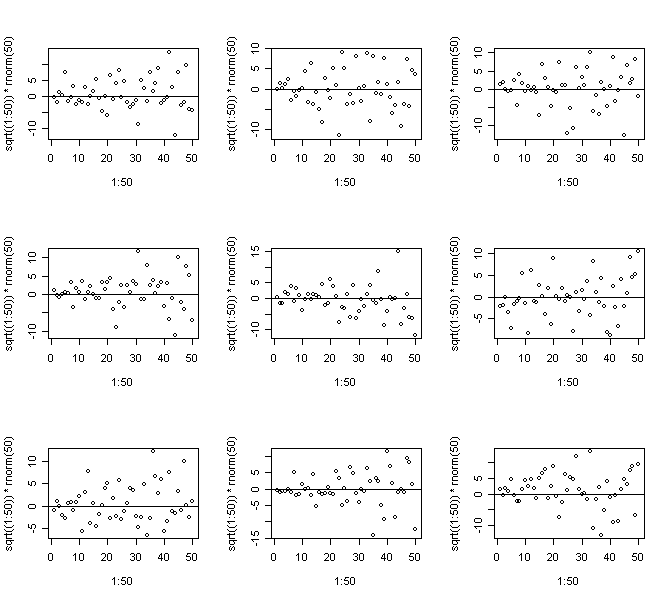> for(i in 1:9) {plot(1:50,cos((1:50)*pi/25)+rnorm(50)); abline(h=0)}  # Non-linearity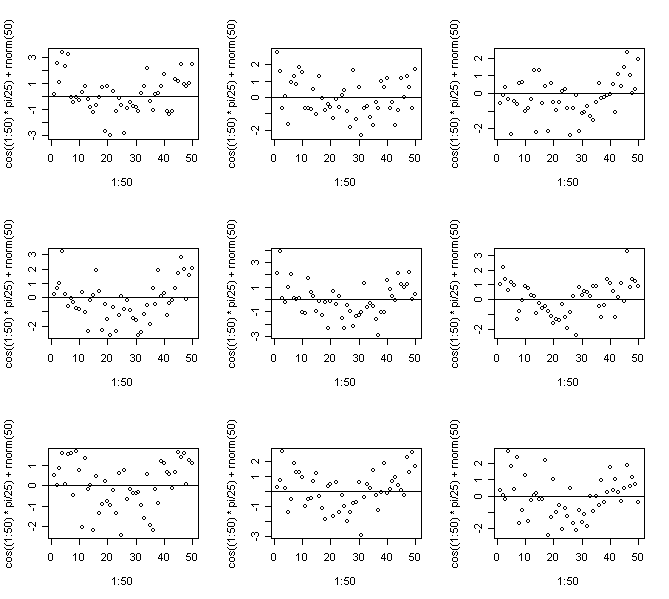• In this case we know the truth - do you think you would be able to come to the right conclusions for real data?

• Repeat to get an idea of the usual amount of variation.

• It's recommended to use the artificial generation of plots as a way to "calibrate" diagnostic plots. It's often hard to judge whether an apparent feature is real or just random variation. Repeated generation of plots under a model where there is no violation of the assumption that the diagnostic plot is designed to check is helpful in making this judgment.

Now let's go back to saving data, and look at the residuals against predictor plots:

> par(mfrow=c(2,3)) # split the graphic window into 2x3 subwindows

> plot(savings\$p15,g\$res,xlab="pop15",ylab="residuals"); abline(h=0) # residual plot - residual against predictor p15
> plot(savings\$p75,g\$res,xlab="pop75",ylab="residuals"); abline(h=0) # residual plot - residual against predictor p75
> plot(savings\$inc,g\$res,xlab="income",ylab="residuals"); abline(h=0) # residual plot - residual against predictor inc
> plot(savings\$gro,g\$res,xlab="growth",ylab="residuals"); abline(h=0)  # residual plot - residual against predictor gro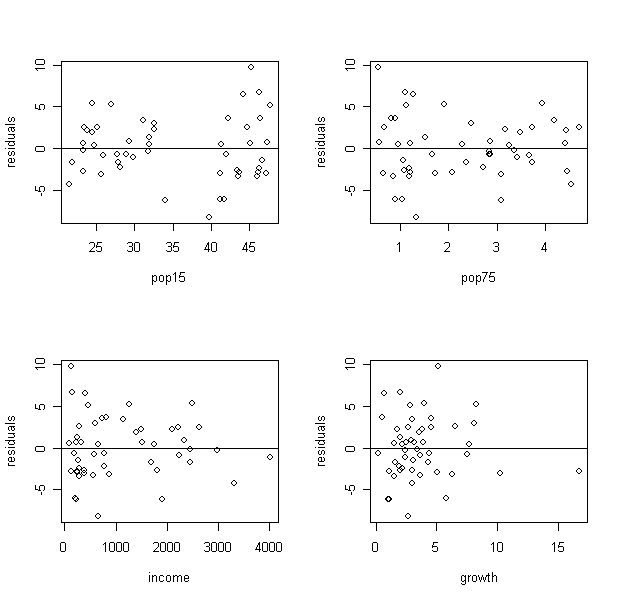• What did you find from the plot of residuals against p15? Can you see the two groups?

• You can compare and test the variance. (Given two independent samples from normal distributions, we can test for equal variance using the test statistics of the ratio of the two variance. The null distribution is a F with degrees of freedom given by the two samples. Try to implement the test by yourself).

• What remedy will you suggest?

• From the plot of residuals against income, what can you find?

• It seems the variance decrease when income increase. Let's further explore it:

> par(mfrow=c(1,1))

> plot(savings\$inc, g\$res, xlab="income", ylab="residuals", type="n") # draw a "no plotting" plot of residual against income

> points(savings\$inc[savings\$p15 <35], g\$res[savings\$p15 <35], pch=1) # label the points with p15<35 as circles

> points(savings\$inc[savings\$p15 >35], g\$res[savings\$p15 >35], pch=4) # label the points with p15>35 as cross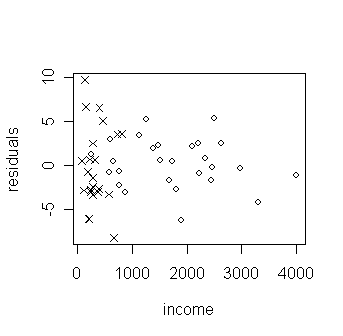• How is the decrease of variance related to the two groups of low and high p15?

A quick way to get various diagnostic plots for the regression is:

> par(mfrow=c(2,2)); plot(g) # some default diagnostic plots in R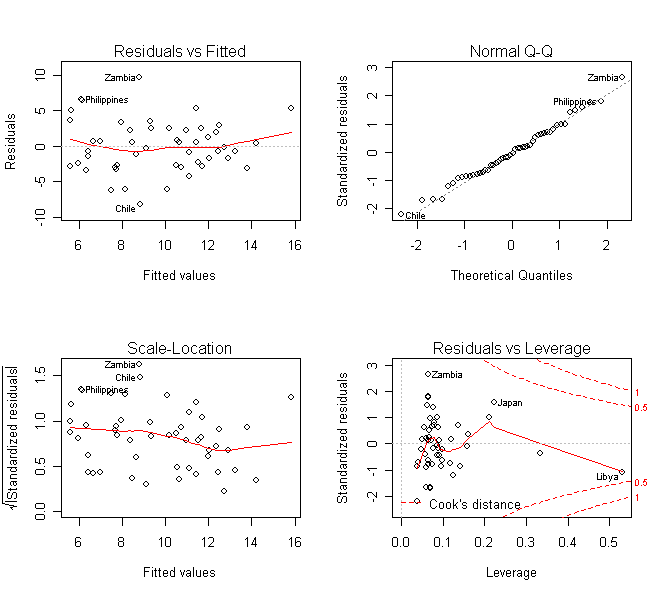# Non-constant variance

Consider the residual vs. fitted plot for the Galapagos data in previous lab.

> gg <- lm(Species~Area+Elevation+Scruz+Nearest+Adjacent, data=gala) # fit a model with Species as response and other variables as predictors

> plot(gg\$fit,gg\$res,xlab="Fitted",ylab="Residuals",main="untransformed response") # draw the plot of residual against y-hat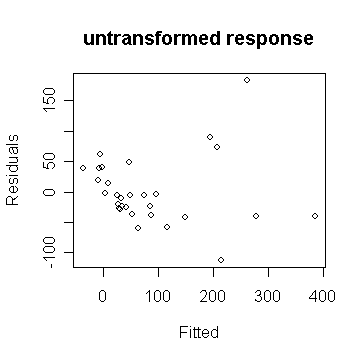• We can observe non-constant variance in the plot.

• From the shape, we guess that a square root transformation (why?) will give us constant variance:

> gs <- lm(sqrt(Species)~Area+Elevation+Scruz+Nearest+Adjacent, data=gala) # fit a model with square root of Species as response and other variables as predictors
> plot(gs\$fit, gs\$res, xlab="Fitted", ylab="Residuals",main="square root response") # draw the plot of residual against y-hat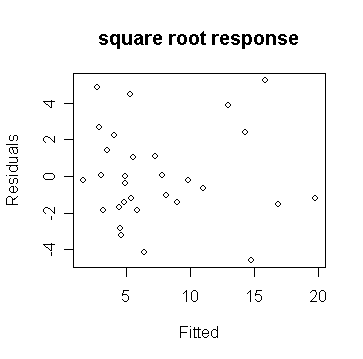• We see that in the 2nd plot, the variance is now constant.

• Our guess at a variance stabilizing transformation worked out here. But, if it's not, we can always try something else.

• The square root transformation is often appropriate for count response data. The Poisson distribution is a good model for counts and that distribution has the property that the mean is equal to the variance, thus suggesting the square root transformation. However, it might be even better to go with a Poisson regression rather than the normal-based regression we are using here.

# curvature in the mean of residuals --- added variable plot and partial residual plot

We use the savings dataset as an example again.

First we construct a added variable plot for p15:

> d <- lm(sav ~ p75 + inc + gro, data=savings)\$res # calculate the residual of the model with sav as response and p75, inc, gro as predictors
> m <- lm(p15 ~ p75 + inc + gro, data=savings)\$res # calculate the residual of the model with p15 as response and p75, inc, gro as predictors
> plot(m, d, xlab="pop15 residuals", ylab="saving residuals", main="added variable plot") # draw the 1st residuals against the 2nd residuals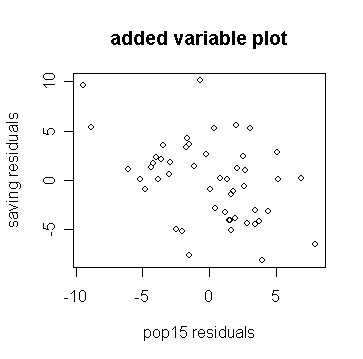• Compare the slope on the plot to the original regression and show the line on the plot:

> lm(d ~ m)\$coef # get the intercept and slope of the fitted line with 1st residual as response and 2nd residual as predictor

(Intercept)         m
-1.231709e-16 -0.461205

> g\$coef # the coefficients of the fitted model with sav as the response and the other variables as predictors

(Intercept)       p15       p75           inc       gro
28.56661 -0.461205 -1.691576 -0.0003367615 0.4096998

> abline(0,g\$coef['p15'])  # add the fitted line to the plot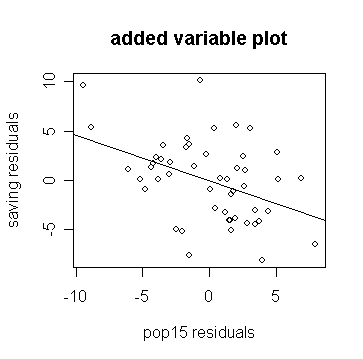• Notice how the slope in the plot and the slope for p15 in the regression fit are the same.

A partial residual plot is easier to do:

> plot(savings\$p15, g\$res+g\$coef['p15']*savings\$p15, xlab="pop15", ylab="saving (adjusted)", main="partial residual") # draw the partial residual plot for p15
> abline(0, g\$coef['p15']) # add the fitted line to the plot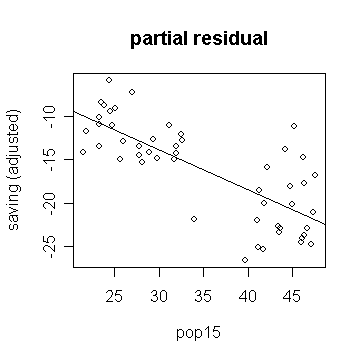or more directly, you can use the function:

> prplot(g,1)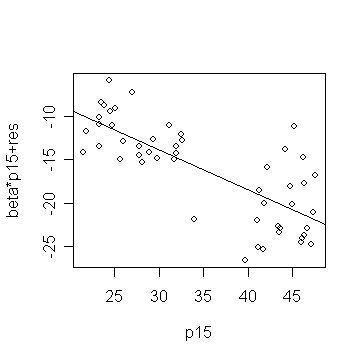• Might there be a different relationship in the two groups:

> g1 <- lm(sav ~ p15 + p75 + inc + gro, subset=(p15 > 35), data=savings) # fit a model for data with p15>35
> g2 <- lm(sav ~ p15 + p75 + inc + gro, subset=(p15 < 35), data=savings) # fit a model for data with p15<35
> summary(g1)  # take a look of the fitted model g1

Coefficients:

Estimate Std. Error t value Pr(>|t|)

(Intercept) -2.4339689 21.1550278  -0.115    0.910

p15          0.2738537  0.4391910   0.624    0.541

p75         -3.5484769  3.0332806  -1.170    0.257

inc          0.0004208  0.0050001   0.084    0.934

gro          0.3954742  0.2901012   1.363    0.190

Residual standard error: 4.454 on 18 degrees of freedom

Multiple R-Squared: 0.1558,     Adjusted R-squared: -0.03185

F-statistic: 0.8302 on 4 and 18 DF,  p-value: 0.5233

> summary(g2) # take a look of the fitted model g2

Coefficients:

Estimate Std. Error t value Pr(>|t|)

(Intercept) 23.9637508  8.0836079   2.964  0.00716 **

p15         -0.3859519  0.1953668  -1.976  0.06089 .

p75         -1.3278580  0.9260337  -1.434  0.16566

inc         -0.0004587  0.0007237  -0.634  0.53271

gro          0.8843841  0.2953329   2.995  0.00668 **

---

Signif. codes:  0 `***' 0.001 `**' 0.01 `*' 0.05 `.' 0.1 ` ' 1

Residual standard error: 2.772 on 22 degrees of freedom

Multiple R-Squared: 0.5073,     Adjusted R-squared: 0.4177

F-statistic: 5.663 on 4 and 22 DF,  p-value: 0.002733

• Can you see the difference? Pay attention to the estimated coefficients of p15 and interpret the difference.

• The graphical analysis has shown a relationship in the data that a purely numerical analysis might easily have missed.

Q-Q plots

Q-Q plots can be used to check the assumptions of normality.

Let's try it out on the same saving data:

> par(mfrow=c(2,2))  # split the graphic window into 2x2 subwindows

> qqnorm(g\$res, ylab="raw residuals") # draw the normal probability plot for raw residuals
> qqline(g\$res) # add a straight line to guide the examination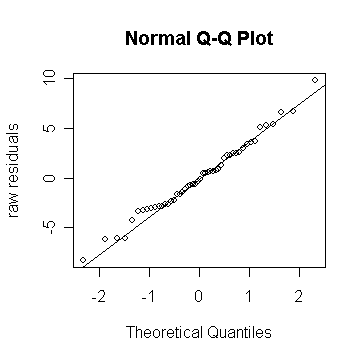• Looks fine - the command "qqline" adds a line joining the first and third quartiles - it's useful as a guide.

We can plot the studentized residuals:

> qqnorm(rstud, ylab="studentized residuals") # draw the normal probability plot for studentized residuals
> abline(0,1) # add the straight line with zero intercept and solpe=1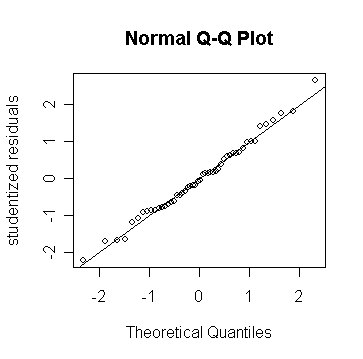• Because these residuals have been normalized, they should lie along a 45 degree line.

Note that histograms and box-plots are not as sensitive for checking normality:

> par(mfrow=c(1, 2)) # split the graphic window into 1x2 subwindows

> hist(g\$res, 10) # a histogram plot for raw residuals
> boxplot(g\$res, ylab="Boxplot of savings residuals") # a box-plot for raw residuals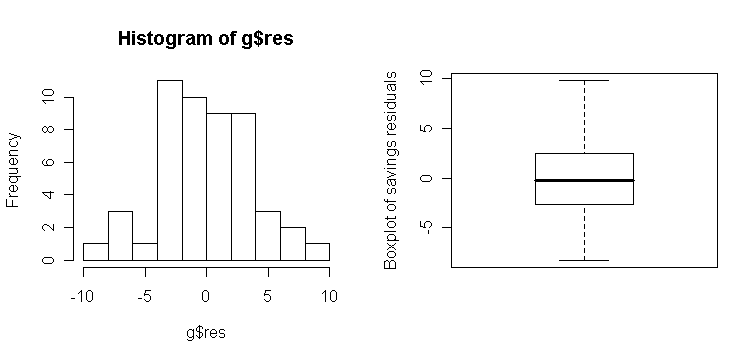We can get an idea of the variation to be expected in Q-Q plots in the following experiment. I generate data from different distributions:

1. Normal

2. Lognormal - an example of a skewed distribution

3. Cauchy - an example of a long-tailed (platykurtic) distribution

4. Uniform - an example of a short-tailed (leptokurtic) distribution

> par(mfrow=c(3,3)) # split the graphic window into 3x3 subwindows
> for(i in 1:9) {x<-rnorm(50); qqnorm(x); qqline(x)} # normal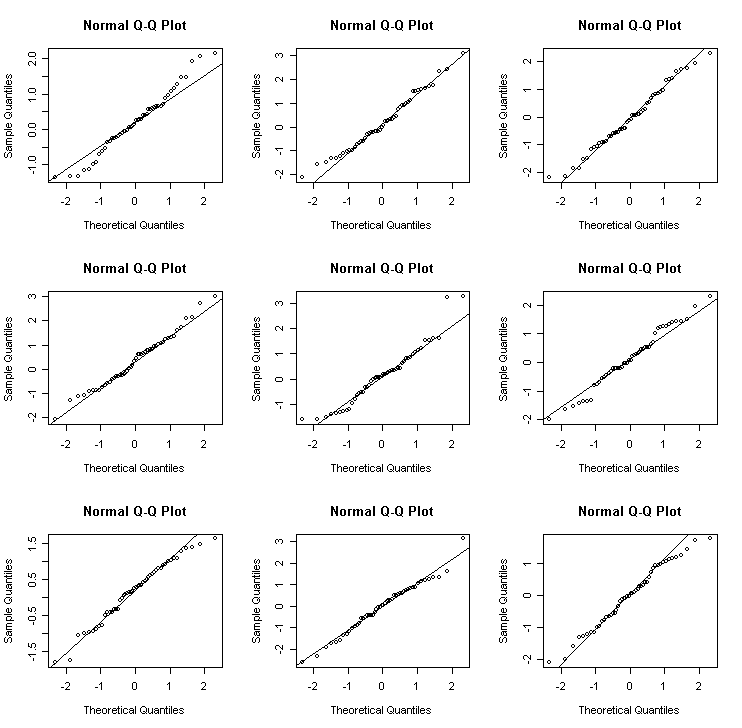> for(i in 1:9) {x<-exp(rnorm(50)); qqnorm(x); qqline(x)} # lognormal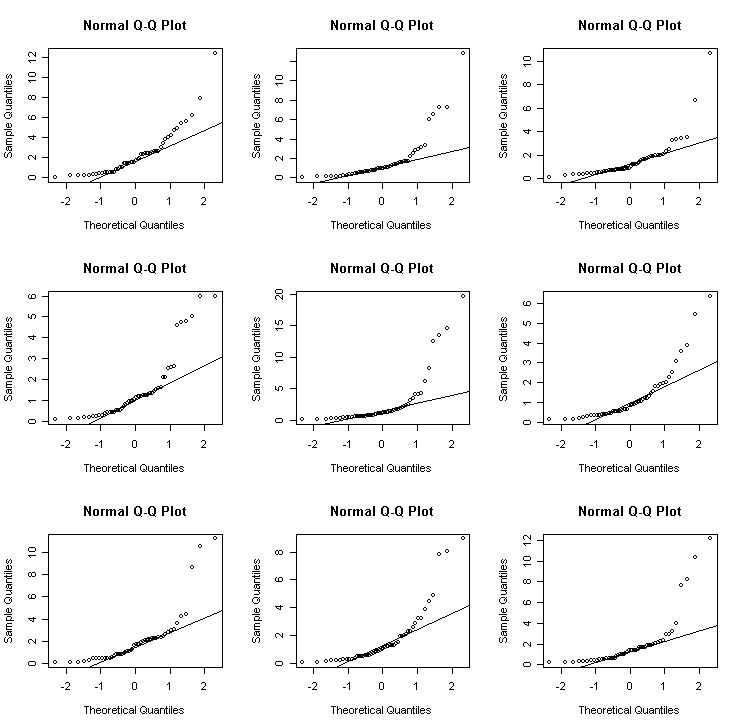> for(i in 1:9) {x<-rcauchy(50); qqnorm(x); qqline(x)} # Cauchy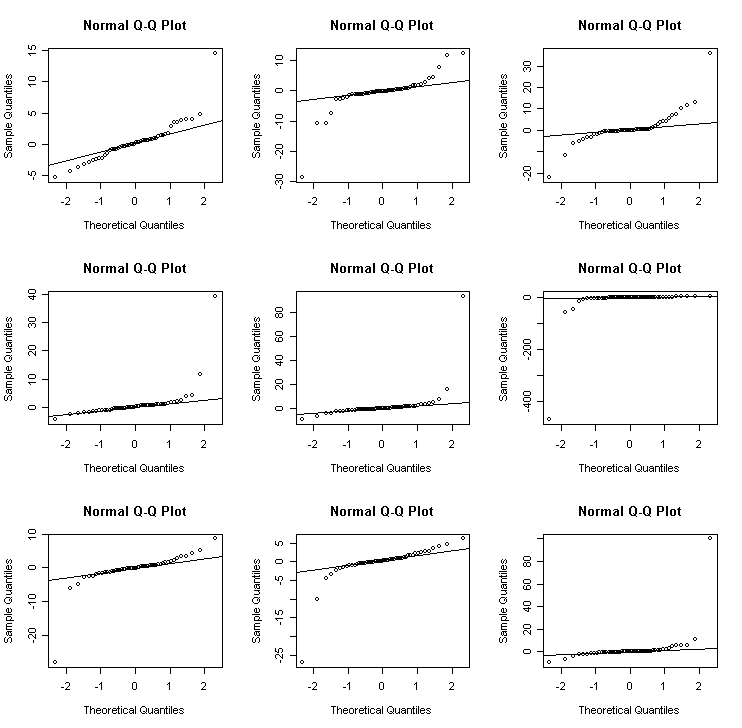> for(i in 1:9) {x<-runif(50); qqnorm(x); qqline(x)} # uniform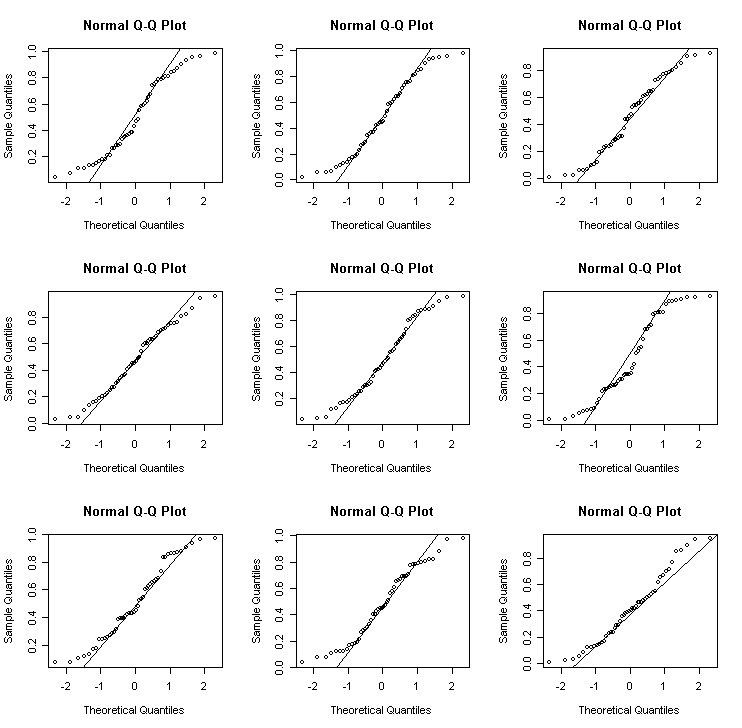Half-normal plot

Half-normal plot is often used to look for extreme values which will be apparent as points that diverge substantially from the rest of the data.

We demonstrate the half-normal plot on the leverages and Cook statistics for the saving data. Here is a function for half-normal plot.

> par(mfrow=c(1,2)); countries <- row.names(savings)

> halfnorm(lm.influence(g)\$hat, labs=countries, ylab="Leverages") # draw half-normal plot for leverage

> halfnorm(cooks.distance(g), labs=countries, nlab=3, ylab="Cook statistics") # draw half-normal plot for Cook's statistics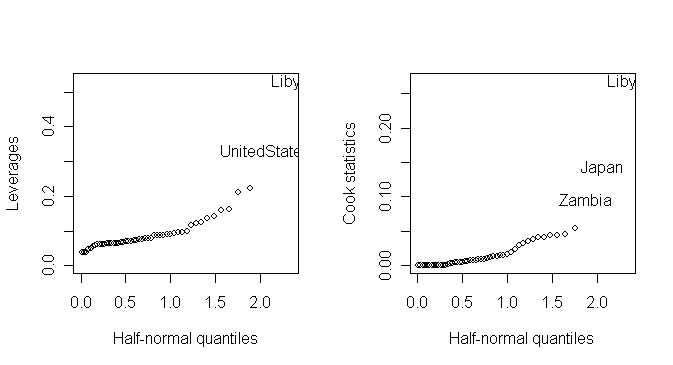Correlated errors

• One of the important assumptions used when fitting the standard linear model is that the errors are uncorrelated.

• When the observations have been collected over time, it is sensible to check for serial correlation.

For the example, we use the data taken from an environmental study that measured the four variables

• ozone,

• temperature, and

• wind speed

for 111 consecutive days.

> air  # take a look of the data

1    3.448217       190          67  7.4

2    3.301927       118          72  8.0

...deleted...

111  2.714418       223          68 11.5

Take a look of the scatter plots of variables in the data set:

> pairs(air) # draw pair-wise scatter plots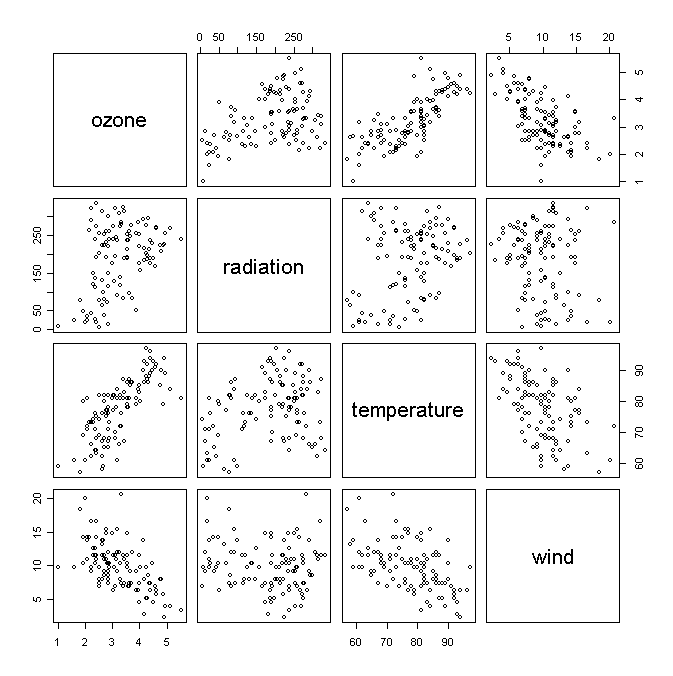We fit a linear model:

> ga <- lm(ozone ~ radiation + temperature + wind, data=air) # fit the model with ozone as response and other variables as predictors
> summary(ga) # take a look of the fitted model

Call:

lm(formula = ozone ~ radiation + temperature + wind, data = air)

Residuals:

Min       1Q   Median       3Q      Max

-1.12160 -0.37635 -0.02535  0.33607  1.49514

Coefficients:

Estimate Std. Error t value Pr(>|t|)

(Intercept) -0.2973296  0.5552139  -0.536 0.593400

radiation    0.0022055  0.0005585   3.949 0.000141 ***

temperature  0.0500443  0.0061062   8.196 5.85e-13 ***

wind        -0.0760219  0.0157548  -4.825 4.67e-06 ***

---

Signif. codes:  0 '***' 0.001 '**' 0.01 '*' 0.05 '.' 0.1 ' ' 1

Residual standard error: 0.5102 on 107 degrees of freedom

Multiple R-Squared: 0.6807,     Adjusted R-squared: 0.6717

F-statistic: 76.03 on 3 and 107 DF,  p-value: < 2.2e-16

Check some diagnostics:

> par(mfrow=c(2, 2)); plot(ga) # draw the default diagnostics plots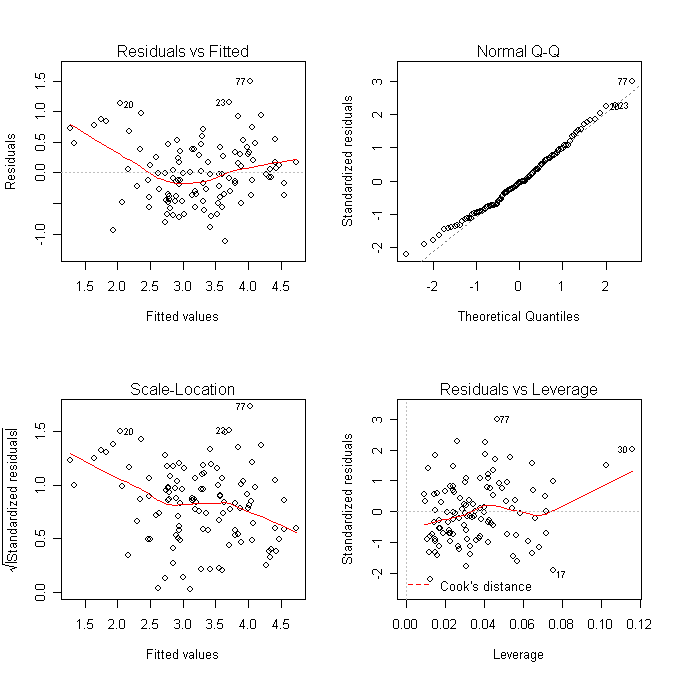• What does the plot of residual against y-hat suggest? Do we need to transform the response?

Suppose we are otherwise satisfied with this model and want to check for serial correlation. First make an index plot of the residuals (note index represents time in this case):

> plot(ga\$res) # draw the plot of residual against index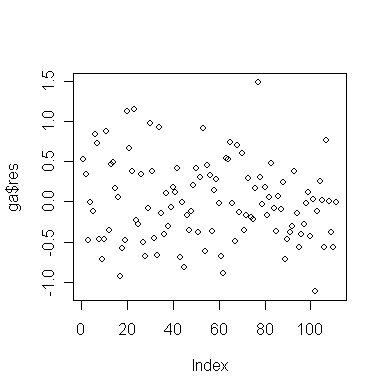• Is there any evidence of serial correlation?

Now plot successive residuals:

> plot(ga\$res[-111],ga\$res[-1])  # plot εt-hat against εt+1-hat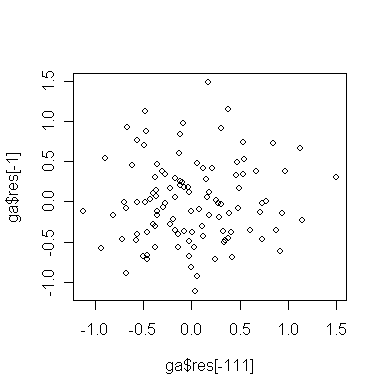• Do you see any problem?

You can plot more than just successive pairs:

> pairs(cbind(ga\$res[-c(109,110,111)], ga\$res[-c(1,110,111)], ga\$res[-c(1,2,111)], ga\$res[-c(1,2,3)]),labels=c("res","lag1 res","lag2 res","lag3 res"))  # plot εt-hat against εt+1-hat, εt+2-hat, εt+3-hat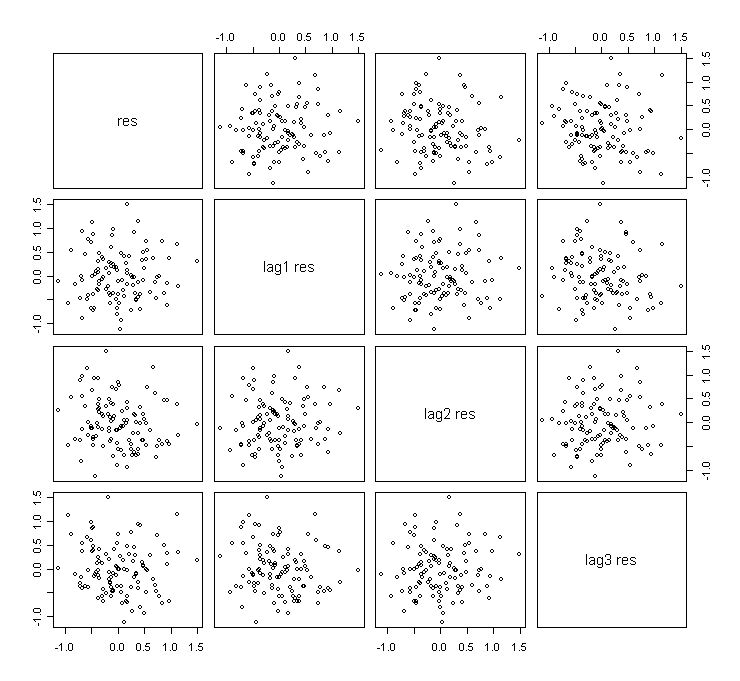• Do you see that some plots are almost identical? Why?

We can compute the Durbin-Watson statistic:

> library(lmtest) # load the "lmtest" library into R

> dwtest(ozone ~ radiation + temperature + wind, data=air) # perform the D-W test

Durbin-Watson test

data:  ozone ~ radiation + temperature + wind

DW = 1.8501, p-value = 0.1893

alternative hypothesis: true autocorrelation is greater than 0

• The p-value indicates no evidence of correlation and note that the D-W statistic is close to 2.